# Ratios and Proportions

Go back to  'Ratio, Proportion, Percentages'

## Introduction to Ratios and Proportions

You want to use orange paint, but you only have red and yellow paints with you. How do you get over this hurdle? Well, you mix the two of course! Orange is nothing but red and yellow paint mixed together, with there being 2 parts of yellow paint for every 3 parts of red paint. That’s what a ratio is!## The Big Idea: What are Ratios?

Simply put, ratios are “The comparison of two values that are parts of a whole.”

Let’s imagine a classroom of 20 students. This class has 5 girls and 15 boys. When asked, what is the ratio of girls to boys in this classroom, you say that it is 5:15 or 5 girls to 15 boys. The sign “ : ” is used to describe a ratio in mathematics.## The Big Idea: What are Ratios and Proportions?

Ratios are a comparison between two numbers (and in some cases even more) that are all components of a much larger whole.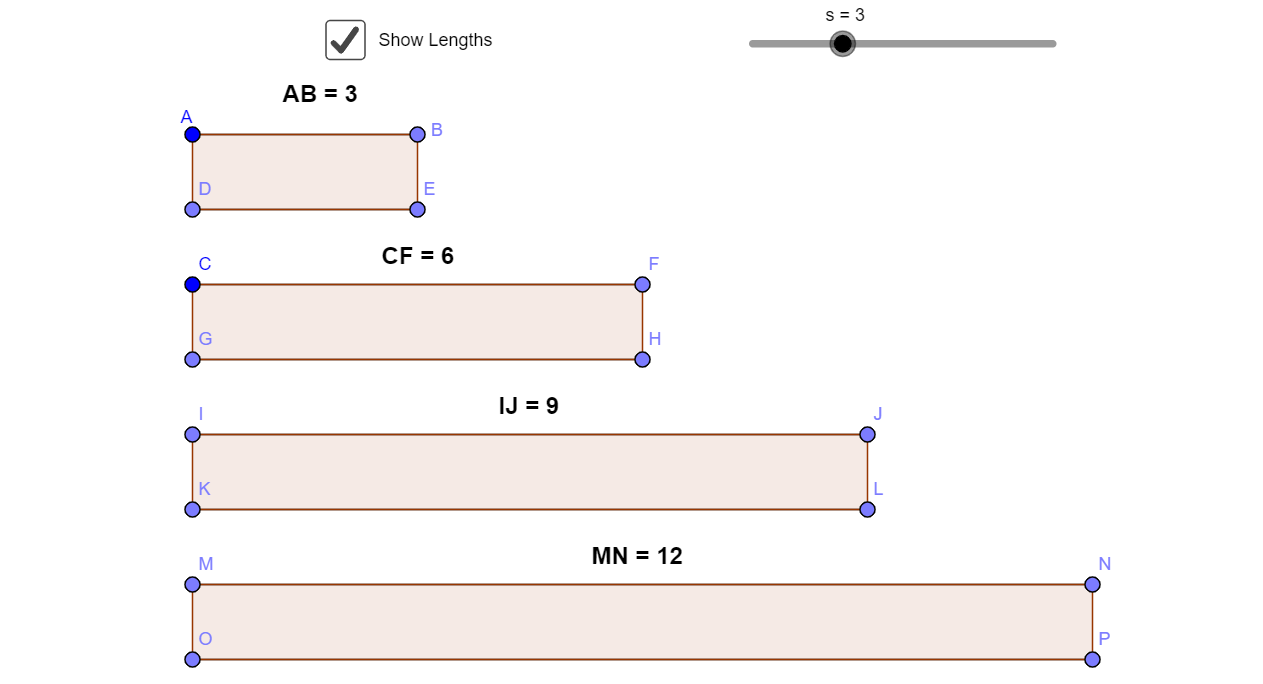While ratios define the relation of each individual component of a whole with other components that make up the same whole, proportions are used to describe how much of each of the components contributes to the whole.

### Constant of Proportionality

Once you’ve mastered the idea of ratios, the next required concept to learn is about the concept of proportionality. From a physical and mathematical standpoint, let’s take the example of speed which can be written as:- Speed = Distance / Time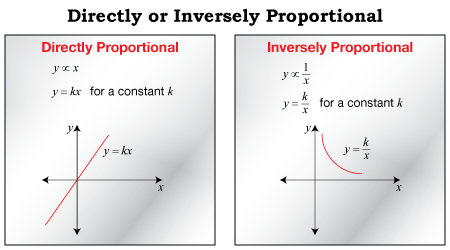It’s important to understand that both sides of the equation must represent parts of the same whole or similar quantities otherwise they won’t be equal.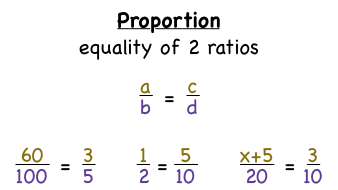Suppose that the speed of a vehicle is to be maintained at a constant value of 100 km/hr. This indicates that in order to maintain the value on the left, any change in distance/time must be met with any corresponding change in the other unit.

Say that the distance and time initially are 100 km and 1 hr respectively. If the distance, however, changes by 120 km. To maintain dimensional consistency, the time would change by 0.833 hr.

In the same manner, if the car takes more time to reach a distance, the distance will too increase to maintain the same 100 km/hr value.

### Ratios and Fractions

Coming to the concept of ratios and fractions, ratios can be further reduced to fractions for better understanding. Taking the example of orange paint again, we know that red and yellow need to be mixed in a ratio of 3:2. That means 3 units of red paint needs to be mixed with 2 units of yellow paint to yield orange paint.

### Components of orangeWhen represented as a ratio, this is what the above image looks like:Remember, that ratios depict the relationship between the components that make up the whole, so the fraction representation of the same ratio is:In the same way, if you had to represent quantities in the context of the whole system, that is to say, how much of red make up orange, that would represent the proportion and would be written in the following manner: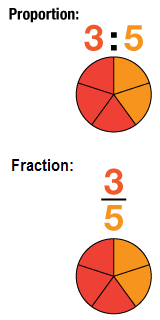Taking a simple example, in a small auditorium that seats 100 people, there are 80 women and 20 men watching a play. While the ratio of women to men will be written as 80:20 or simplified, 4:1 as for every 4 women there is 1 man present, the proportions are 80:100 or 4:5 for women, and 20:100 or 1:5 for men.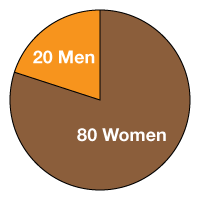Have a look below to get a glimpse of different ways of visualising ratios and proportions: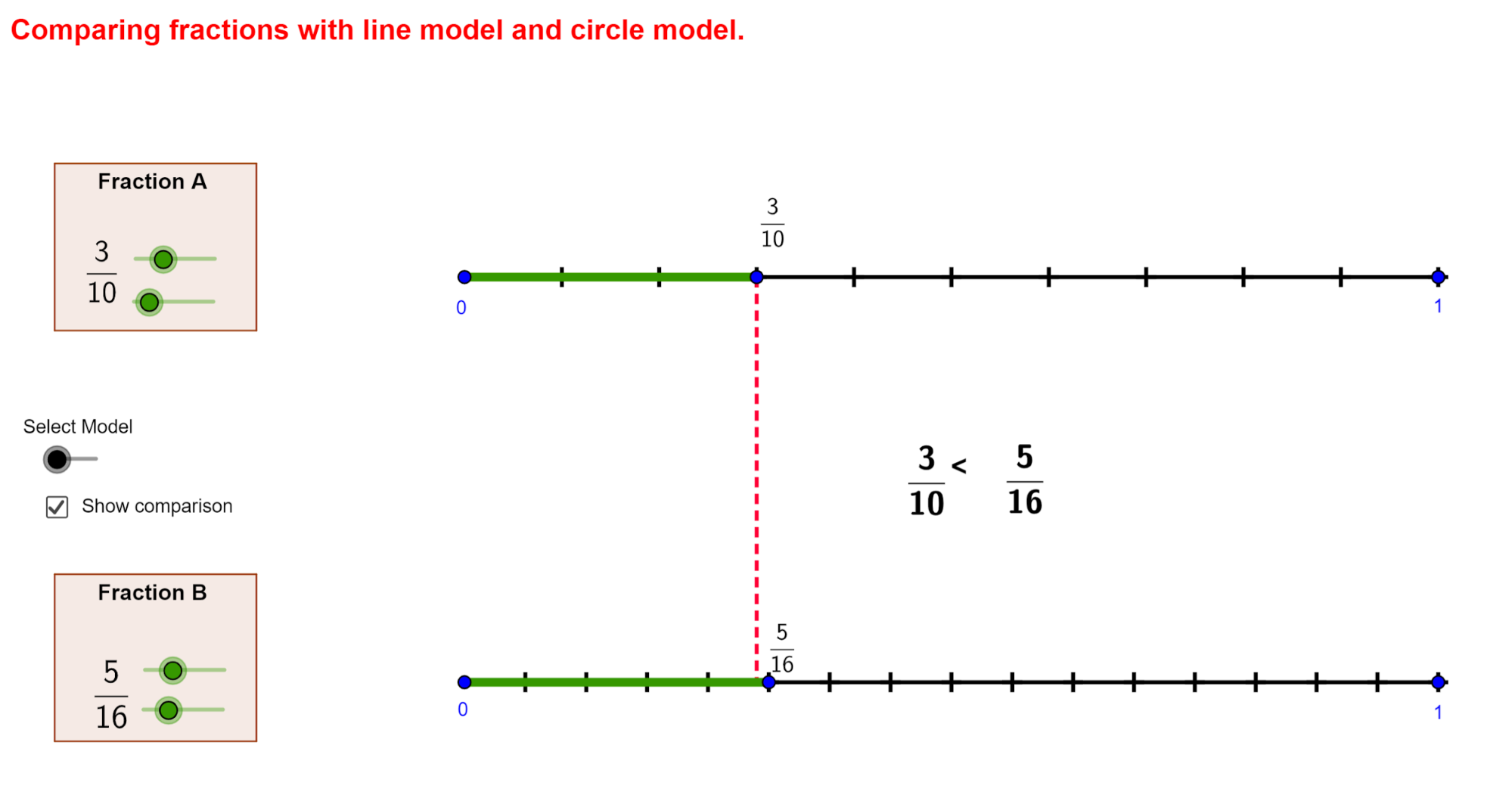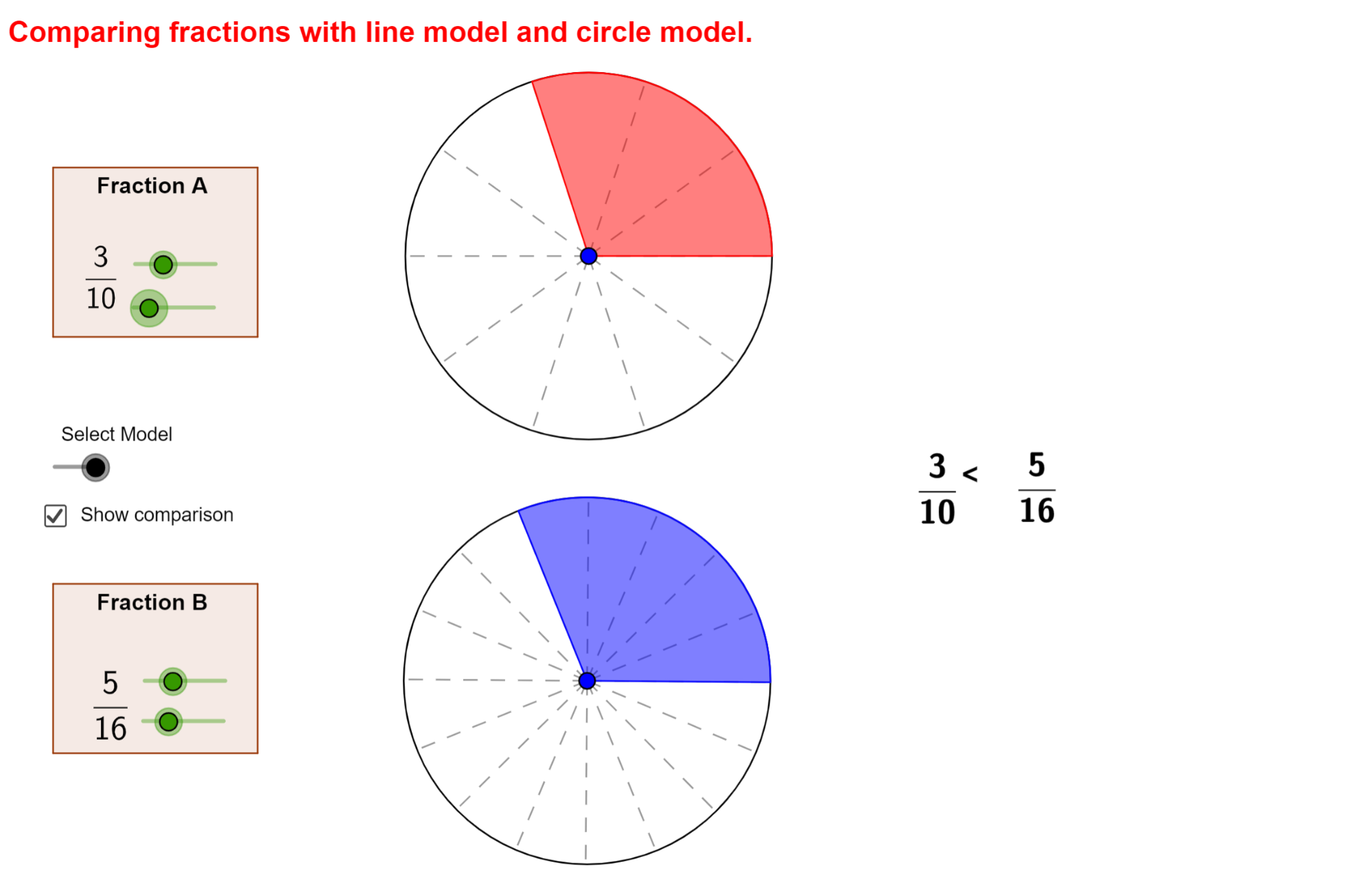### What are the Basic Doubts that Arise While Reading About Ratios?

One of the biggest doubts that arise with fractions come with quantities that take more than two ratio components.

Ratios like 5:6:7 and 1:5:8:10 can be difficult to grasp at first but are not so tough to understand. The best way to do this is by converting them into fractions.

For starters, always remember that the denominator value would be the same of all the individual quantities. The individual fractions would be arrived at by dividing the values with the total sum.

Ratios like 3:7:5 can thus be represented as $${3 \over 15}$$, $${7 \over 15}$$ and $${5 \over 15}$$ respectively.

Another major doubt regarding ratios arises when comparing two or more different ratios with a parameter that connects them.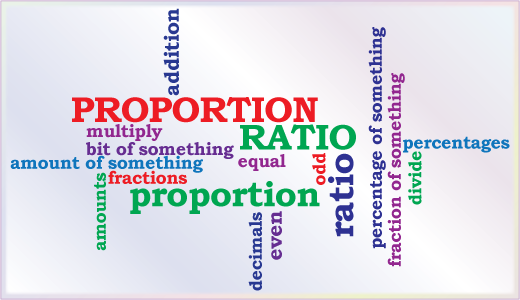For example, think of two lots of goods filled with apples and bananas (5:7) and another filled with bananas and oranges (3:4). Suppose you’re questioned to find the ratio of apples to oranges. The conventional method would be to take the LCMs for both the ratios and then equating them to come up with a final ratio.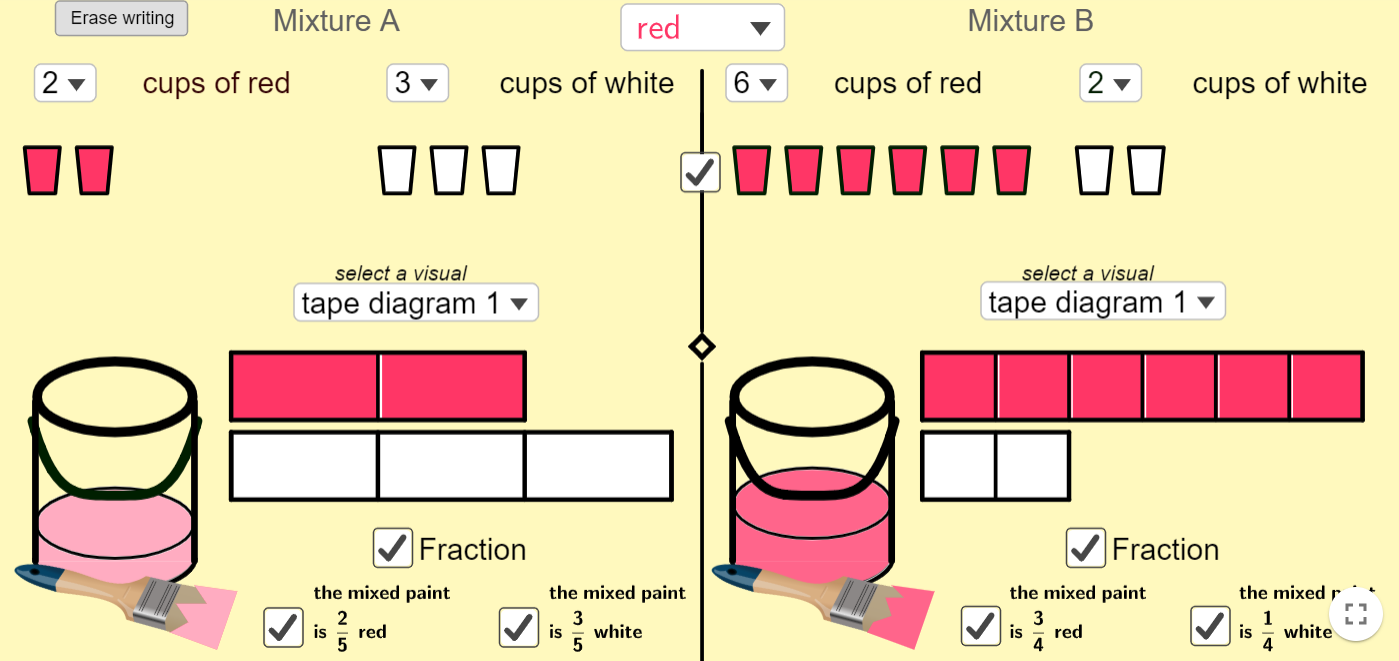The simpler method is to simply equate the quantities that are common by a common factor and then eliminate them both.

For example, taking the above case:-

(5:7)*3 gives 15:21 and (3:4)*7 gives 21:28.
By eliminating 21, you get 15:28 which is the required quantity.

Another common doubt is about proportions that are equivalent but with a quantity missing, say for example, 6:4 = x:2 where x is an unknown quantity.

Instead of wasting time by taking up common LCMs, simply cross multiply the known values to arrive at x which in this case is 3.

Always remember that for proportions, numerator values are proportionate to the main quantity on the left while denominator values are inverse in effect.

This indicates that keeping the main quantity constant, increase in the numerator will result in an increase in the denominator. In the same way, any decrease in the numerator will result in a decrease in the denominator to keep both sides proportionate.